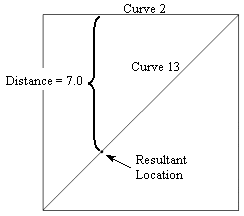# Specifying a Location on a Curve

Some commands require you to specify a location on a curve (i.e., webcutting with a plane normal to a curve). The following are the options for specifying a location (or locations in the case of the segment option) on a curve:

## Center

center

The center option helps in identifying the location at the center of a given arc. Example: create vertex center curve 3

## Start, Midpoint, or End

{ MIDPOINT | Start | End |

These options simply specify the location that is the midpoint, start or end point of a curve. By default, the midpoint is the understood location unless another location is specified.

## Fraction

Fraction <val 0.0 to 1.0> [From Vertex <id> | Start|End] |

The fraction option simply finds the location that is a fractional distance along the curve. By default, the fraction references the start of the curve; however, you can optionally specify which vertex to reference from.

## Distance

Distance <d> [From {Vertex|Curve|Surface} <id> | Start | End ] |

The distance option not only can find a location that is a certain distance along the curve from the start or end of the curve, but can also find a location (or locations if there is more than one solution) on a curve that is a specified distance from another curve or a surface. If the From Curve option is used both curves must lie in the same plane.

draw location on curve 13 distance 7 from curve 2Figure 1 - Location on a Curve a Distance from Another Curve

## {Close_To|At} Location

{{Close_To|At} Location {options} | Position <xval><yval><zval> |{Node|Vertex} <id>} |

These options take a location closest to the location on the curve.

## Extrema

Extrema [Direction] {options} [Direction {options}] [Direction {options}]

The extrema option finds the maximum value location along a curve in a specified direction. For example:

create vertex location on curve 1 extrema ny

Creates a vertex on curve 1 at the location where the y axis value of the curve is at a minimum.

## Segment

Segment <num_segs>

The segment option finds locations spaced evenly along the curve such as to break the curve into equal length "segments" (of course the curve is not modified). You must specify a minimum of two segments (if two segments were specified a location would be found at the center of the curve). The following example results in 4 locations:

draw location on curve 1 segment 5

create vertex on curve 1 segment 5Figure 2 - Five Segments on a Curve

## Crossing

Crossing {Curve|Surface} <id_list> [Bounded|Near]}

The crossing option finds locations at the intersection of the curve and another curve or surface. By default, the curve(s) and surface are extended to infinity and the intersections are calculated; if the bounded option is specified only intersections that lie on the bounded entities will be returned. The near option is valid only for two linear curves. If near is specified the nearest location between the two linear curves will be returned.

## Previewing a Location on a Curve

A location on a curve can be previewed with the Draw command. All of the options that can be used for specifying a location on a curve can be used to preview a location on a curve. See above for a description of these options. The command syntax is:

Draw Location On Curve <curve id> {options}